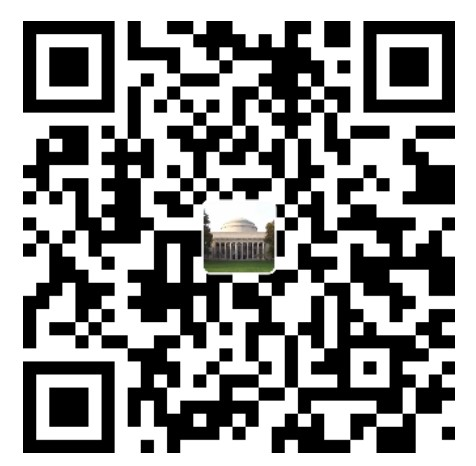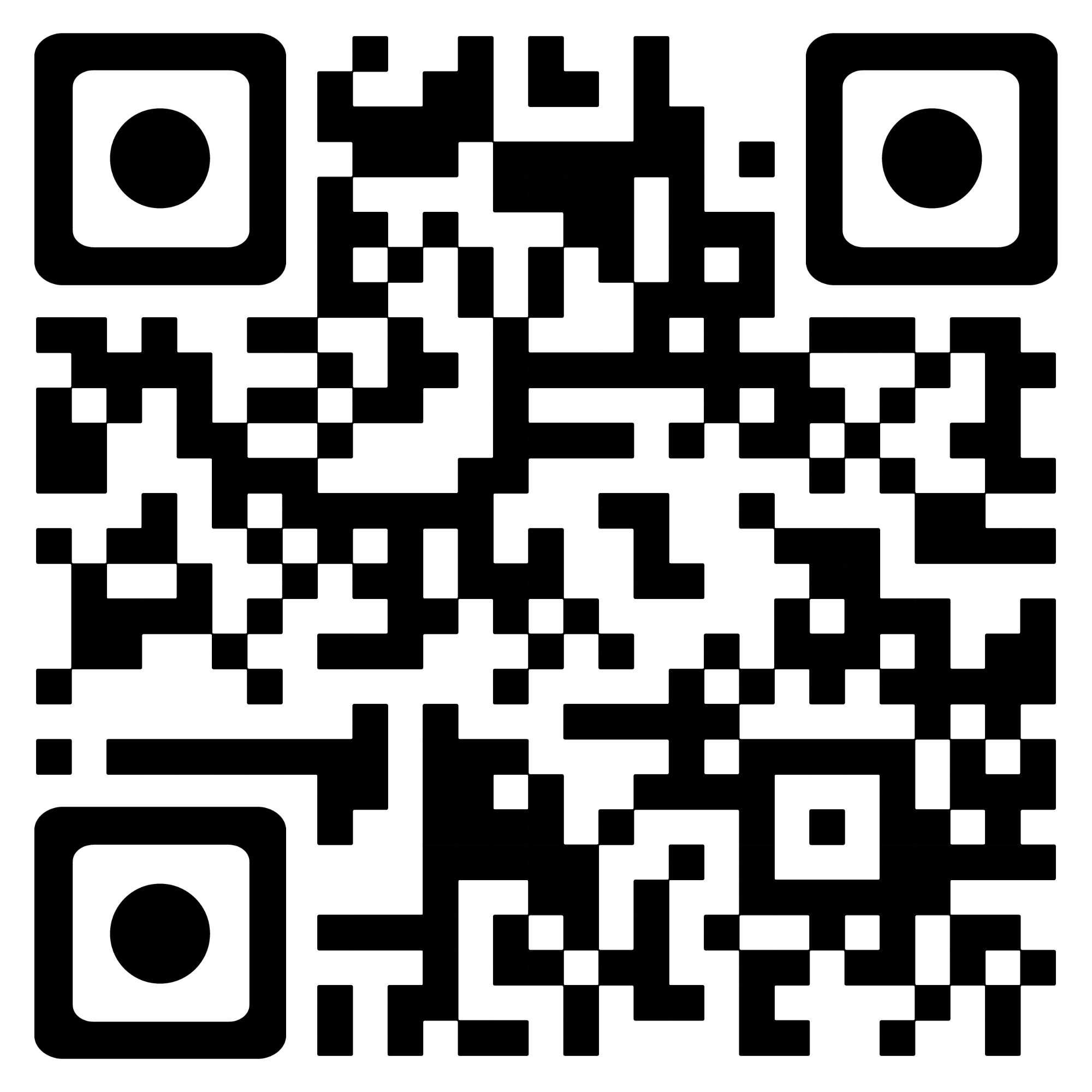Permutation in String

# Desciption

Given two strings s1 and s2, return true if s2 contains a permutation of s1, or false otherwise.

In other words, return true if one of s1's permutations is the substring of s2.

Input: s1 = "ab", s2 = "eidbaooo"
Output: true
Explanation: s2 contains one permutation of s1 ("ba").

Slide Window

# Code

• submit code
``````class Solution:
def checkInclusion(self, s1: str, s2: str) -> bool:
need_dict = {}
need_count = 0
# 需求字典创建
for i in s1:
need_dict[i] = need_dict.get(i,0) + 1
need_count += 1

window_list = {}

right = 0
left = 0
valid = 0

while right < len(s2):
c = s2[right]
right += 1

if(need_dict.get(c,0) > 0):
window_list[c] = window_list.get(c,0) + 1
if(window_list.get(c) == need_dict.get(c)):
valid += 1

while(right - left >= len(s1)):
if(valid == len(need_dict)):
return True

d = s2[left]
left += 1

if(need_dict.get(d)):
if(need_dict.get(d) == window_list.get(d,0)):
valid -= 1
window_list[d] = window_list.get(d) - 1

return False
``````
• full code
``````class Solution:
def checkInclusion(self, s1: str, s2: str) -> bool:
need_dict = {}
# 需求字典创建
for i in s1:
need_dict[i] = need_dict.get(i,0) + 1

window_list = {}

right = 0
left = 0
valid = 0

while right < len(s2):
c = s2[right]
# print("c"+c)
right += 1

if(need_dict.get(c)):
window_list[c] = window_list.get(c,0) + 1
if(window_list.get(c) == need_dict.get(c)):
valid += 1

while(right - left >= len(s1)):

if(valid == len(need_dict)):
return True

d = s2[left]
left += 1

if(need_dict.get(d)):
if(need_dict.get(d) == window_list.get(d,0)):
valid -= 1
window_list[d] = window_list.get(d) - 1

return False

S = Solution()
# s1 = "ab"
# s2 = "eidbaooo"
s1 = "abcdxabcde"
s2 = "abcdeabcdx"

print(S.checkInclusion(s1,s2))
``````

### 要不赞赏一下?微信支付宝PayPalBitcoin

``https://www.emperinter.info/2022/02/26/permutation-in-string/``